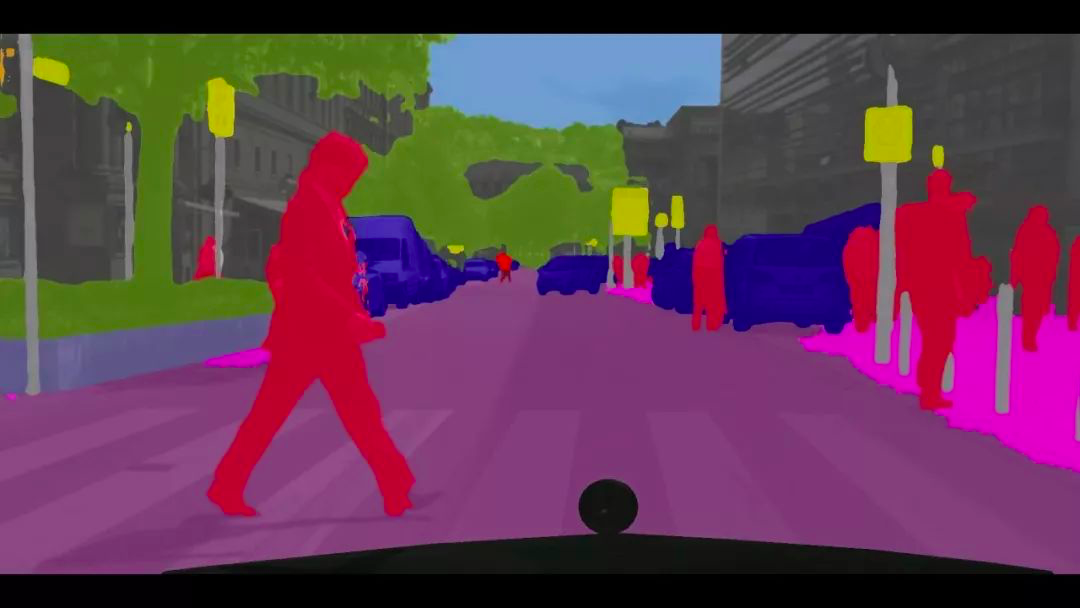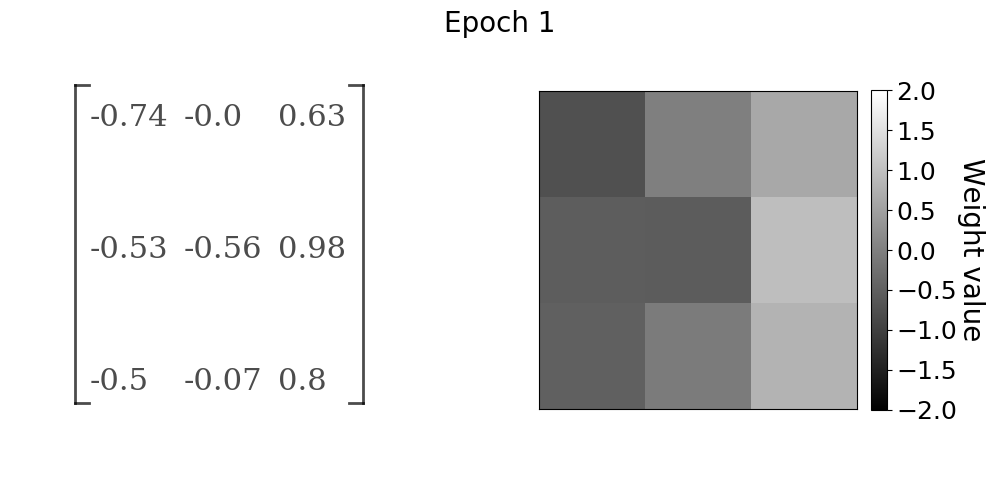Covers: theory of convolutions
Estimated time needed to finish: 16 minutes
• Why to use Convolutions?
• What is an image filter?
• What is image segmentation and how is it done?
• What convolution does really do?
• What are two famous and edge detection filters / algorithms?
##### How to use this item?

In this video you will learn about:

• Why to use Convolutions?
• What is image segmentation and how is it done?
• What convolution does really do?
• Edge detection: Sobel Operator
• Edge detection: Scharr Operator

#### Why to use Convolutions?

For data with hig dimensionality, for example images (3D but 2D for every RGB channel), but also time-series, a single input for a NN may be respresented by thousands of data points. Specifically, a gray image of dimensionality 224×224 contains over 50K pixels! Imagine image classification task. Would each pixel in the image be correlated with the subject/class in the picture? Rather, the networks should itterativelly be trained to associate particular areas of the image capturing at least some part od the subject/class .

#### What is image segmentation and how is it done?

Look at the image bellow! Segments or objects, if you want, are masked with different colors. E.g. people - red mask, cars - blue mask? How it is done? By convolution! If you are interested to learn more about segmentation, you may be interested in Mask R-CNN, one of the image segmentation state-of-the-art model.#### What convolution does really do?

First, it reduces dimensionality of the input data, so called down-pooling! It uses the kernel operator - a matrix - sliding on the input data. By learning kernel weights it extracts properties in the data/image like edges.

### What is an 'image filter' (Edge detection)?

It's the kernel! ! The sliding matrix on the matrix (data). In other words, it is a matrix applied to an image in order to recognize patterns.e.g. you can highlight edges applying a sobel filter through matrix multiplicationFail to play? Open the link directly: https://youtu.be/7MzPOxL7zlk
Amir Hajian
0 comment
Recipe
publicShare
Star(0)

### Extracting Features Using Convolution And Regularization

ContributorsTotal time needed: ~4 hours
Objectives
Feature extraction via convolution kernels
Potential Use Cases
Building a DL architecture for for computer vision model.
Who is This For ?
INTERMEDIATE
Click on each of the following annotated items to see details.
Resources6/7
VIDEO 1. Mathematics of Convolution
• Why wee need more than MLP?
• What is a convolution and kernel?
• What is a Hann function and how to apply it?
• What are ConvNets and what is their architecture?
31 minutes
VIDEO 2. Why Convolutions? Sobel & Scharr Filters
• Why to use Convolutions?
• What is an image filter?
• What is image segmentation and how is it done?
• What convolution does really do?
• What are two famous and edge detection filters / algorithms?
16 minutes
VIDEO 3. 2D Convolutions, Pooling, and Dilated Convolutions
• What is pooling and padding?
• What other 2D convolution techniques do exist?
• When do we use padding and how?
• When do we use pooling and how?
• What are dilated convolutions and how they differ from standard convolutions?
30 minutes
VIDEO 4. Conv-Nets
• How can I apply Sobel and Scharr Operator on image?
• What is the difference between Sobel and Scharr Operator and how can I visually compare them?
• What is a Convolutional Neural Network (CNN)?
• How can one understand a CNN?
20 minutes
VIDEO 5. Regularization using Dropouts
• Why do we need dropouts?
17 minutes
REPO 6. Hands-on Convolutional Networks
• How to implement convnets in PyTorch?
30 minutes
RECIPE 7. Understanding Convolution
40 minutes

0 comment